# Writing Percents as FractionsProblem: One team won 18 out of every 25 games, and another team won 72% of all games played. Which team has a better winning record?

We know from previous lessons that a percent is a ratio whose second term is 100. So we get the following:

72% = 72 out of 100 =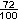and

18 out of 25 ==Solution: Both teams have the same winning record.

Any percent can be written as a fraction in lowest terms. One method for reducing a fraction to lowest terms is to divide both the numerator and the denominator by their greatest common factor (GCF). Let's look at an example of this.

Example 1: Write each percent as a fraction in lowest terms:    55%,    41%,    36%

 Solution Percent Fraction Reducing Lowest Terms 55%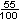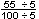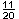41%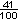36%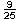In Example 1, the GCF of 55 and 100 is 5; the GCF of 41 and 100 is 1; and the GCF of 36 and 100 is 4. Note that when the GCF is 1, this means that the fraction is already in lowest terms. Let's look at some more examples.

Example 2: Write each percent as a fraction in lowest terms:    7%,    12.5%,    62.5%

 Solution Percent Fraction Reducing Lowest Terms 7%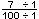12.5%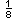62.5%Example 3: Write each percent as a fraction in lowest terms:    67.5%,    56.25%,    13.1%

 Solution Percent Fraction Reducing Lowest Terms 67.5%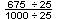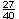56.25%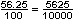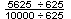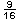13.1%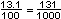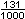Summary: To write a percent as a fraction in lowest terms, follow these steps:

1. Write the percent as a fraction with a denominator of 100.
2. Reduce the fraction to lowest terms.

### Exercises

Directions: Read each question below. Select your answer by clicking on its button. Immediate feedback is provided in the RESULTS BOX. If you make a mistake, choose a different button.

 Which of the following is equal to 90%?None of the above.       RESULTS BOX:
 2.  Which of the following is equal to 16%?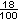None of the above.       RESULTS BOX:
 3.  Which of the following is equal to? .7272%       All of the above.       RESULTS BOX:
 4.  Which of the following is equal to .875? 8.75%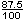None of the above.       RESULTS BOX:
 5.  Which number has the greatest value? .625       6.25%       \$6.25       6,250 cents       RESULTS BOX: